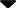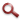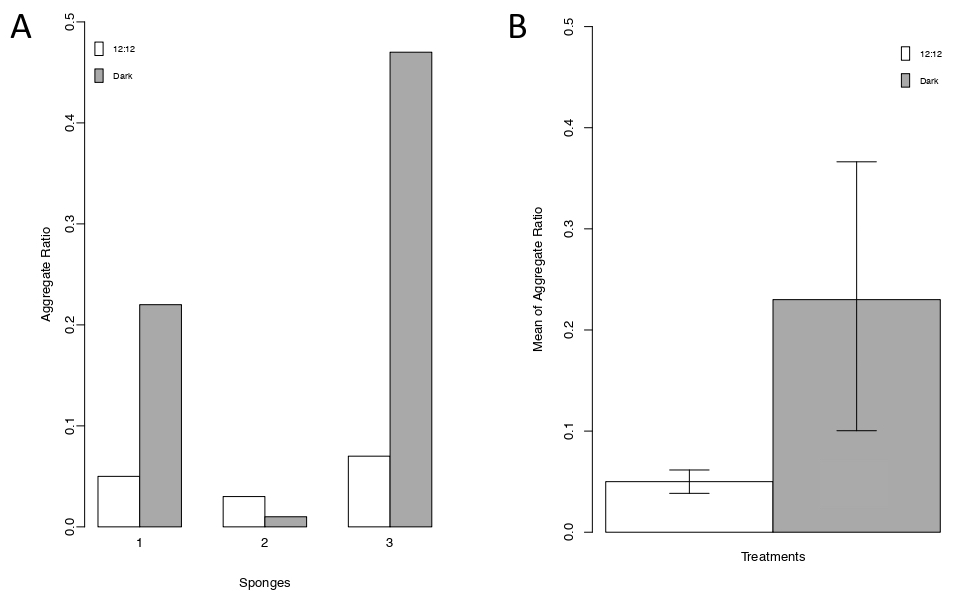Site WebYou are here:

## Student Project FiguresFigure 4 Figure 4 Aggregate ratio of all samples. (A). Aggregate Ratio of Big over small aggregates ratio for each A. queenslandica after 7 day of reaggregation process. Light colour stands for the 12:12 light:dark treatment. Dark colour stands for the constant dark. (B) Mean of aggregate ratio from all sample in both treatments (Mean ± SEM). Figure 2 Figure 3 Figure 4 Figure 5 Figure 6